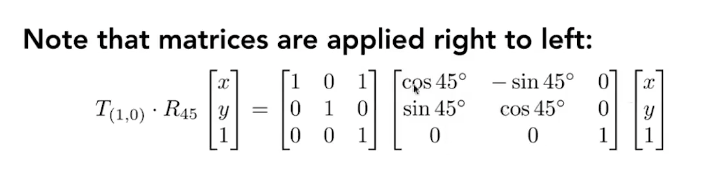# 计算机图形学-01

## 2D和3D点的表示### 2D点旋转与移动

``````constexpr double PI = 3.1415926535;

double angle = 45;
double radian = angle * PI / 180.0f;
Eigen::Vector3f p(2.0f, 1.0f, 1.0f);    // 点(2,1)

// 旋转
Eigen::Matrix3f r3;
0, 0, 1;

// 位移
Eigen::Matrix3f m3;
m3 << 1, 0, 1,
0, 1, 2,
0, 0, 1;

p = r3 * p;     // 先逆时针旋转45度

p = m3 * p;     // 再位移(1,2)
``````

### 3D旋转

``````[cos(45),-sin(45),0,0,
sin(45),cos(45),0,0
0,    0,    1,    0,
0,    0,    0,    1]
``````

Star 0
BACK